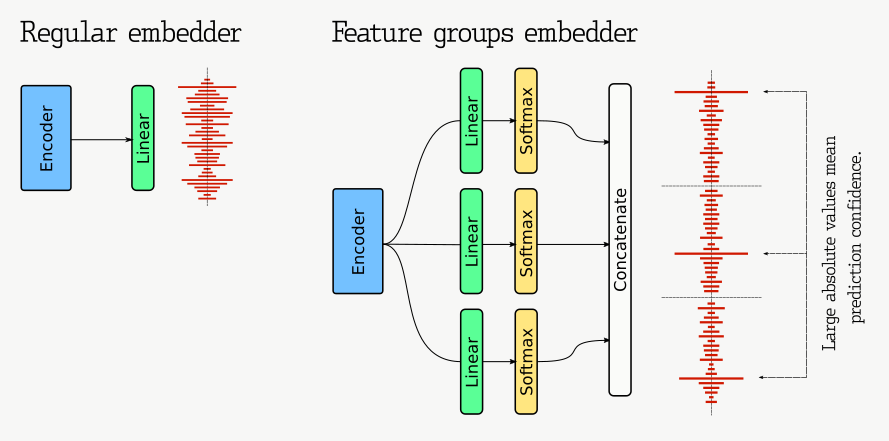Shortcuts

# Embedding Confidence¶

In real life, knowing how confident the model was in the prediction is often needed. It is helpful to know if manual adjustment or validation of the result is required.

With conventional classification, it is easy to understand by scores how confident the model is in the result. If the probability values of different classes are close, the model is not confident. If, on the contrary, the most probable class differs significantly, then the model is confident.

At first glance, we cannot apply this technique to similarity learning. Even if the predicted object similarity score is small, it might only mean that the reference set has no proper objects to compare. Conversely, the model can group garbage objects with a large score.

Fortunately, there is a small modification to the embedding generator, which allows you to define confidence in the same way as it is done in conventional classifiers with a Softmax activation function.

The modification consists in building an embedding as a combination of feature groups. Each feature group is presented as a one-hot encoded sub-vector in the embedding. If the model can confidently predict the feature value — the corresponding sub-vector will have a high absolute value in some of its elements. We recommend thinking about embeddings not as points in space but as a set of binary features for a more intuitive understanding.To implement this modification and form proper feature groups, we would need to change a regular linear output layer to a concatenation of several softmax layers. Each softmax component would represent an independent feature and force the neural network to learn them.

Let’s take, for example, 4 softmax components with 128 elements each. Every such component could be roughly imagined as a one-hot-encoded number from 0 to 127. Thus, the resulting vector will represent one of 128⁴ possible combinations. If the trained model is good enough, you can even try to interpret the values of singular features individually.

class Model(TrainableModel):
...

output_groups=4,
output_size_per_group=128,
input_embedding_size=input_embedding_size
)


Discover Qdrant

## Similarity Learning

Explore practical problem solving with Similarity Learning

Learn Similarity Learning

## Community

Find people dealing with similar problems and get answers to your questions

Join Community